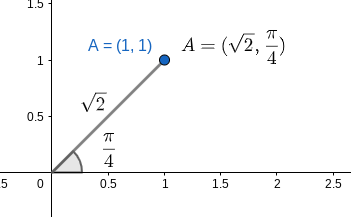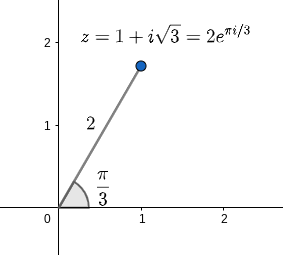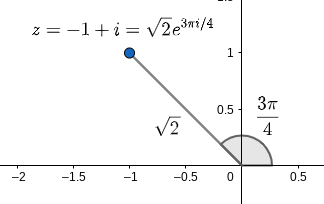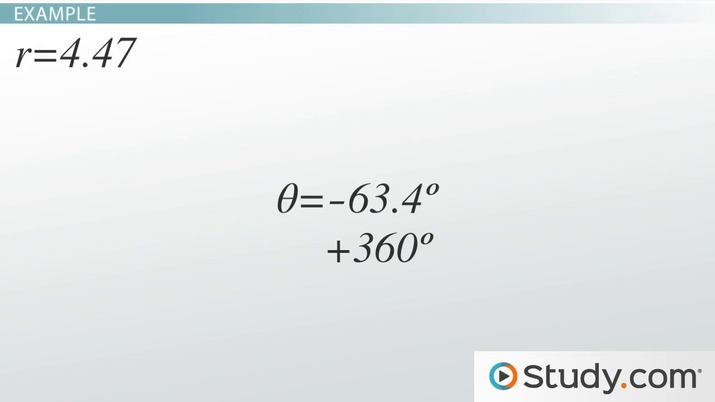# Complex Numbers in Polar Form: Computation and Examples

Emma May, Yuanxin (Amy) Yang Alcocer
• Author
Emma May

Emma May is a mathematician with a bachelor's degree in mathematics from Vassar College. She has tutored subjects such as calculus, linear algebra, and multivariable calculus for over three years. She also conducted mathematics research in topics such as combinatorics and dynamics for over four years.

• Instructor
Yuanxin (Amy) Yang Alcocer

Amy has a master's degree in secondary education and has been teaching math for over 9 years. Amy has worked with students at all levels from those with special needs to those that are gifted.

The polar form of a complex number is an alternative way to write a complex number. The polar form is easy to compute. Examples are provided. Updated: 12/29/2021

Show

## The Polar Form of Complex Numbers

A complex number is a number with an imaginary component. The imaginary component is represented by i, which is the square root of -1. A typical complex number in standard form looks like a + bi, where a and b are real numbers. The real part is a, and the imaginary part is b.

The real plane has two dimensions. A point on the real plane is written in Cartesian form as an ordered pair (x,y), where x and y are real numbers. The real plane can also be represented through polar form. In polar form, a point is represented as (r,x) where r is the radius, which is the distance from the point to the origin, and x is the angle formed between the point, the origin, and the x-axis. For this lesson, all angles will be given in radians. For example, consider the point (1,1) in Cartesian coordinates. This can be converted into polar form by taking the tangent inverse of the y-coordinate over the x-coordinate. Thus, {eq}\arctan\frac{1}{1} = \arctan 1 = \frac{\pi}{4} {/eq}. Then, the radius is the distance from the origin to (1,1). This is {eq}\sqrt{1^2 +1^2} = \sqrt{1+1} = \sqrt{2} {/eq}. Hence, the point in Cartesian form (1,1) is equivalent to the point ({eq}\sqrt{2} {/eq}, {eq}\frac{\pi}{4} {/eq}) in polar form.Complex numbers both have a Cartesian-like form and a polar form as well. A complex number in standard form has a real part and an imaginary part, which serve as its coordinates on the complex plane. Similar to the real plane, the standard form of a complex number may be turned into polar form.

### How To Write Complex Numbers in Polar Form

To write a complex number in polar form given its standard form, there are a few easy steps. Let z = a + bi be a complex number.

• Take the modulus of z, which is its magnitude. This is calculated via {eq}|z| = |a + bi| = \sqrt{a^2+b^2} {/eq}.
• Find the angle formed between z and the real line. This is {eq}\theta = \arctan\frac{b}{a} {/eq}. Remember to use the appropriate {eq}\theta {/eq} given by its position on the complex plane. If z is in the third or fourth quadrants, then make sure that {eq}\theta {/eq} corresponds to that quadrant.
• Write the complex number in polar form. This is {eq}z = |z|(\cos \theta + i\sin\theta) = |z|e^{i\theta} {/eq}.

It is important to note here that {eq}e^{i\theta} = \cos\theta + i\sin\theta {/eq}. This is identity will not be proven in this lesson, but it is important to know.

To go from the polar form to standard form, do {eq}|z|e^{i\theta} = |z|(\cos\theta +i\sin\theta) = |z|\cos\theta + |z|i\sin\theta {/eq}.

### Example 1: Converting Complex Numbers to Polar Form

Consider the complex number {eq}z = 1 + i\sqrt{3} {/eq}. To turn it into a polar form, first, take its modulus. This is {eq}|z| = \sqrt{1^2 +(\sqrt{3})^2} = \sqrt{1 + 3} = \sqrt{4} = 2 {/eq}. Then, find the angle between z and the real line: {eq}\arctan\frac{\sqrt{3}}{1} = \arctan \sqrt{3} = \frac{\pi}{3} {/eq}. Last, put the two pieces together to find that {eq}z = |z|e^{i\theta} = 2e^{\pi i/3} {/eq}.### Example 2: How To Convert Complex Numbers to Polar Form

Consider the complex number given in standard from z = -1 + i. To write this in polar form, first take the modulus of the complex number: {eq}|z| = \sqrt{(-1)^2 + 1} = \sqrt{1+1} = \sqrt{2} {/eq}. Then, find the angle between z and the real line: {eq}\arctan\frac{1}{-1} = \arctan (-1) = \frac{3\pi}{4} {/eq}. Last, combine the two pieces to find that {eq}z = -1 + i = \sqrt{2}e^{3\pi i/4} {/eq}.An error occurred trying to load this video.

Try refreshing the page, or contact customer support.

Coming up next: Evaluating Parametric Equations: Process & Examples

### You're on a roll. Keep up the good work!

Replay
Your next lesson will play in 10 seconds
• 0:01 Complex Numbers
• 0:56 Polar Form Conversion
• 2:37 Example
• 4:18 De Moivre
• 6:20 Lesson Summary
Save Save

Want to watch this again later?

Timeline
Autoplay
Autoplay
Speed Speed

To unlock this lesson you must be a Study.com Member.

#### What is the polar form of a complex number?

The polar form of a complex number replaces the standard form by turning it into a product of its radius of the number with e to the power of i theta, where theta is the angle formed between the complex number and the real line.

#### How do you write a complex number in polar form?

Suppose a complex number is a + bi. First, find its modulus which is the square root of the sum of a squared plus b squared. Then, find the angle by doing the arctan of b over a. Then, write the two together as the modulus of z times e to the power of i theta, where theta is the angle found in step 2.

### Register to view this lesson

Are you a student or a teacher?